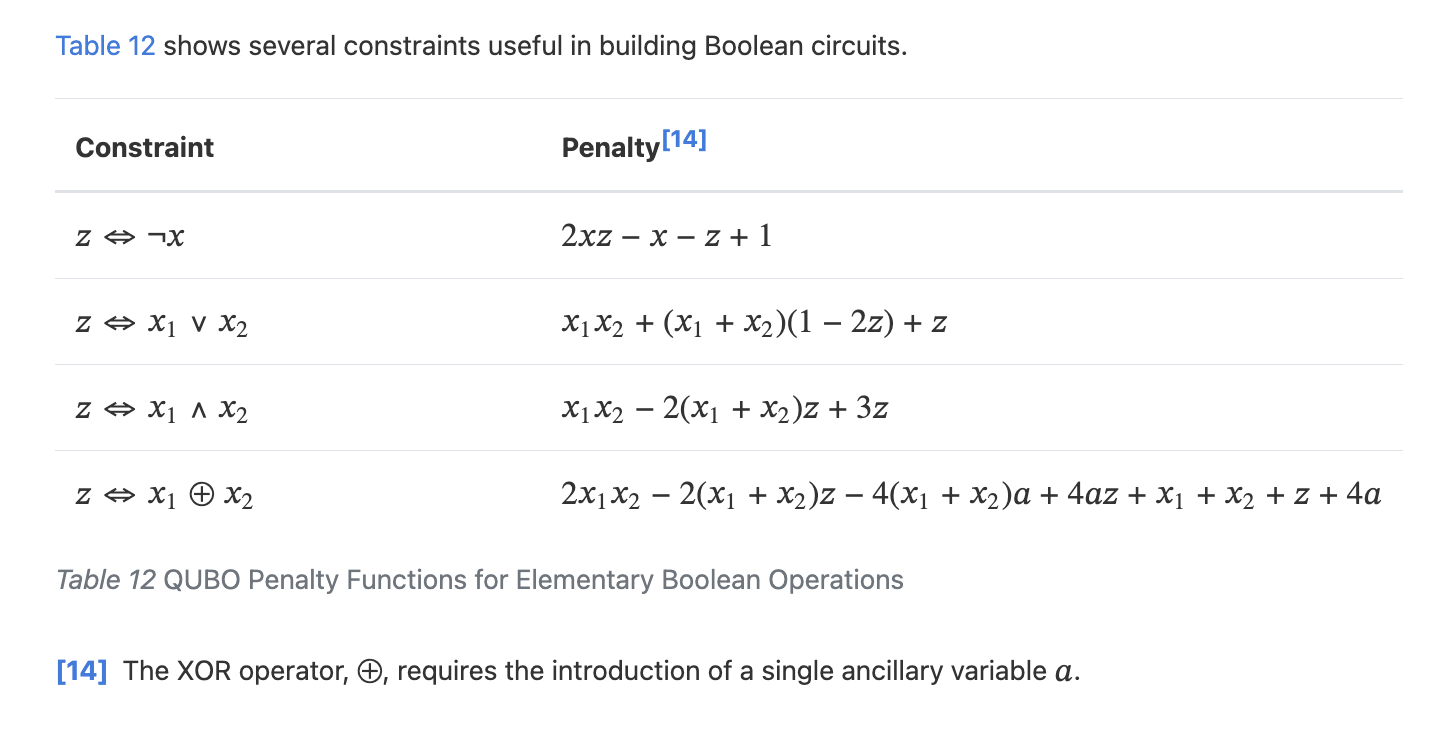# XOR parity constraint as a QUBO

Dear Community,

I just started learning about formulating problems for quantum annealing. I'm interested in realizing a parity constraint as a QUBO problem. A parity constraint requires the number of 1s in a set of variables to be either even or odd. For example, a XOR b = 1 (the set {a,b} must have an odd number of ones) or a XOR b XOR c = 0 (the set {a,b,c} must have an even number of ones).

In the Problem Solving Handbook, I came across the following table:It was upsetting to see that an XOR operator requires an ancillary variable because the XOR parity constraints I am interested in are very long.

Just to be sure, I tried formulating a BQM for x_1 XOR x_2 = 1 by making a constraint satisfiability table. I got 1 - x_1 - x_2 + 2*x_1*x_2. I thought that maybe you don't need ancillary variables.

But then I tried finding a QUBO equation for x_1 XOR x_2 XOR x_3 = 1. I was unable to find the equation because a cubic term is required to create an equation that works for all input combinations. I hypothesize that for an n-variable parity constraint, you need n-2 ancillary variables, to compensate for the lack of cubic, quartic, and higher-order polynomial terms in QUBO. This might be why z <=> x_1 XOR x_2 required an ancillary bit (because there are three variables).

I'm wondering how to realize x_1 XOR x_2 XOR x_3 = 1 and ultimately, n-variable parity constraints, as a QUBO with ancillary variables. I don't really understand the how ancillary variables work conceptually (in a QUBO). Any resources are appreciated. I was also wondering if there are ways to reduce the number of ancillary variables as their number may get very high if my (n-2) hypothesis is correct. My end goal is to realize a system of parity constraints as a QUBO and optimize the number of 1s outputted as a solution using quantum annealing.

I am a total beginner so it is almost guaranteed that I am misunderstanding something really basic here. I would really appreciate any articles or tutorial suggestions about this stuff.

Many thanks!

1 comment
• Hello,

There are a few places in the documentation that you can learn about how ancillary variables are used.
Doing a quick search for "ancillary" in the following pages might be helpful:

Also, using the search bar at the top of the page in the Leap Community and Help Centre or this page will give you access to related resources within our documentation, community, and publications:

Often creating a truth table or penalty model for a smaller problem is the most effective way to understand what is possible within the constraints of a particular use case. The above reformulation handbook does a good job of demonstrating this.

Hopefully this was helpful. Please feel free to reach out with further questions.